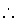Next: Ordinary Differential Equations Up: Small Projects Previous: The Blocks World

# A Discussion on DONALD + GERALD = ROBERT

This puzzle, similar to SEND + MORE = MONEY, consists of trying to find out integer values between 0 and 9 so that the arithmetical operation

 D O N A L D + G E R A L D R O B E R T

makes sense. We will follow an approach similar to that of SEND + MORE = MONEY, but we will use Prolog IV, which will allow us showing how enumeration predicates can be built upon simpler ones. This will also show us how to use interval arithmetic in order to simulate finite domains, using the appropriate primitive constraints. We will as well explore how choosing the order of variables and the right primitive to enumerate will affect the total time of the search.

Our first program is as follows:

dgr(X):-
X = [D,O,N,A,L,G,E,R,B,T],
allintin(X, 0, 9),
gt(D, 0),
gt(G, 0),
all_diff(X),
100000.*.D .+. 10000.*.O .+. 1000.*.N .+. 100.*.A .+. 10.*.L .+. D .+.
100000.*.G .+. 10000.*.E .+. 1000.*.R .+. 100.*.A .+. 10.*.L .+. D =
100000.*.R .+. 10000.*.O .+. 1000.*.B .+. 100.*.E .+. 10.*.R .+. T,
enumlist(X).


Recall the SEND + MORE = MONEY program in Section 1.7, and pay attention to the similarities. Since we want to simulate FD with interval arithmetic, we are using the non-linear, intervals version of the arithmetic operations. Some predicates called in the code before have to be defined by the user (they are not directly available in Prolog IV, but their definition is not difficult):

allintin/3
expresses that all variables in the list of the first argument have integer values which are between a minimum and a maximum (the second and third arguments):

allintin([], _Min, _Max).
allintin([X|Xs], Min, Max):-
int(X),
ge(X, Min),
ge(Max, X),
allintin(Xs, Min, Max).


all_diff/1
imposes the constraint that all elements in the list must be different. This is programmed using the dif/2 builtin which forces two terms to be different:

all_diff([]).
all_diff([X|Xs]):-
diffs(X,Xs),
all_diff(Xs).

diffs(_X, []).
diffs(X, [Y|Ys]):-
dif(X,Y),
diffs(X, Ys).


enumlist/1
performs a enumeration of the elements in the list by enumerating the variables in the order of the list.
enumlist([]).
enumlist([X|Xs]):-
enum(X),
enumlist(Xs).


This program takes 134.3 seconds to solve the problem.7.1 Of course, this is quite high--but, on the other hand, the program is really simple. We may try to improve the performance of the program by making a smarter selection of order of enumeration of the variables: a feasible heuristic, as mentioned in Section 5.2, is enumerating first the most constrained variables. Simply counting how many times a variable appears in the main equation of the problem allows us to sort the list of variables in this order: [D, R, O, A, L, E, N, G, B, T], where variables which appear more often go first. Setting X = [D, R, O, A, L, E, N, G, B, T] at the beginning of the program cuts the execution time down to 28.2 seconds. Reversing this order ([T, B, G, N, E, L, A, O, R, D]) increases the execution time to 36.8 seconds, which suggests that the most-constrained ordering of variables is not necessarily the winner, since the less-constrained order is not as bad as the quasi-random one we chose first. Trying new orderings, and seeing which ones make sense, would need an auxiliary tool to help understand how constraint solving behaves; these tools, usually graphical displays of the constraint solving, exist, but it is not a task of this introductory paper dealing with them. We will try two new variable orderings, in the hope that some light is shed on the direction of the optimal search path.

First we will try the order [D, T, L, R, A, E, N, B, O, G], i.e., enumerating the variables as they appear in a right-to-left column-by-column traversal of the operation (as it is done when making the addition by hand). The result is all but encouraging: this time finding the solution takes 369 seconds. The reverse ordering, ([G, O, B, N, E, A, R, L, T, D]), is surprisingly good: the puzzle is solved in 15.8 seconds. A feasible explanation is that, since the leftmost digits are the ones which have more height in the whole operation, a wrong selection will be detected before. This may be true, but it is only partially exemplified in the ordering selected: due to the removing of duplicates in the list, the order of less significant digits before'' reversed is not most significant digits before'', but less significant digits after''. The most significant digits before'' is actually [D, G, R, O, E, N, B, A, L, T], and using this enumeration order the execution time is lowered to a better mark of 7.1 seconds.

Usually other enumeration primitives are available. In Prolog IV the builtin intsplit/[1,2,3] performs a dynamic, intelligent (and, if desired, user-programmed) selection of the variable to be enumerated next. Using it does not help to achieve better results with the last ordering, which seems to be quite good, but it does help with other orderings: the results with the third ordering proposed ([T, B, G, N, E, L, A, O, R, D]) results in an execution time of 1.1 seconds, although the time to explore the whole execution tree is quite high.It is possible to write an alternative set of constraints for the problem: instead of coding directly the desired equation, the manual carry-based algorithm can be coded as a set of equations. Carry is generated for each column, and added from the previous column:

dgr(X):-
X = [D, T, L, R, A, E, N, B, O, G],
Carry = [C1, C2, C3, C4, C5],
allintin(X, 0, 9),
gt(M, 0),
gt(S, 0),
allintin(Carry, 0, 1),
all_diff(X),